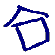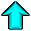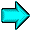# Buckling of long thin axially compressed shells

Want to use light and strong structures and predict when they will fail.

The elastic buckling of long thin cylinders is a classic problem in structural mechanics. It has long been recognized experimentally that the buckle pattern occurs locally along the axial length of the cylinder. However the standard classic approach assumes that the buckle pattern comprises of an interaction of periodic eigenmodes axially and circumferentially. In our work we treat the buckling as a localization phenomenon.

The most widely used model for the buckling of thin cylinders rests with the von Karman-Donnell equations:governing radial displacement w and in-plane stress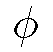.

The parameters appearing are the curvature rho := 1/R,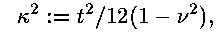where nu is Poisson's ratio and the bifurcation parameterwhere P is the compressive axial load applied per unit length and E is Young's modulus. The equations are supplemented with periodic boundary conditions in the circumferential direction and asymptotic boundary conditions in the axial direction.

We discretize in the circumferential direction by a Galerkin method to get a nasty looking system of ODEs.
Here are the first 6 Fourier modes for the displacement and stress. We clearly see the localized nature of the solution. The modes are shown at a minimum load. This was found by numerical continuation using the code auto. A typical bifurcation diagram looks like thisHere we have reconstructed the solution over the cylinder for the displacement w (on left) and the stress(on the right or below)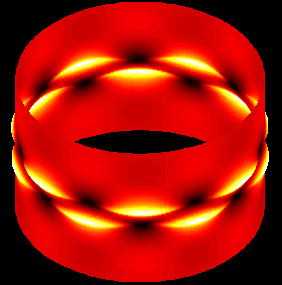A large AVI Movie